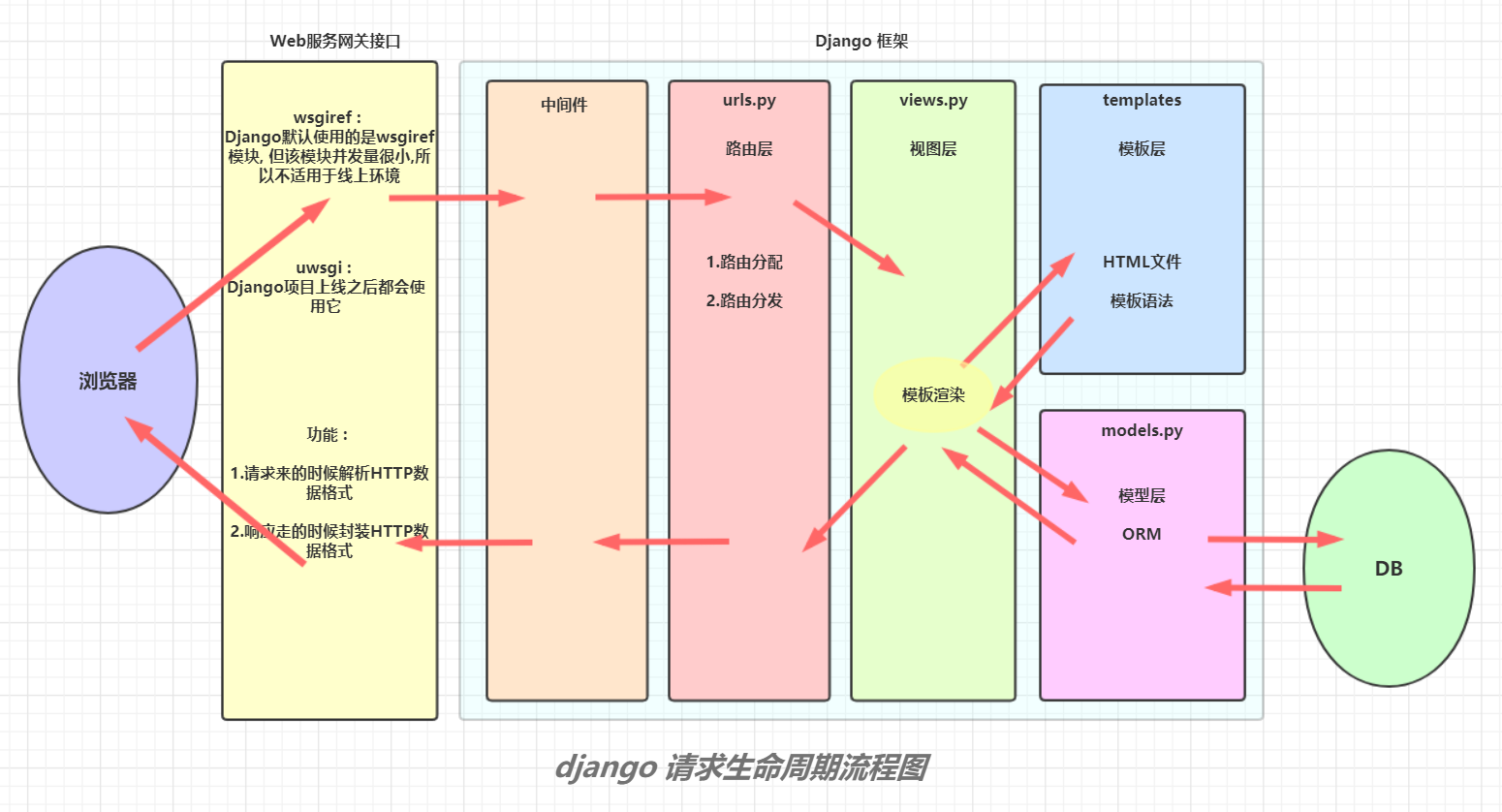# 07 django ORM 表关系与django 请求生命周期流程图

82次阅读

## 一. 表与表之间的关系

• 一对多
• 一对一
• 多对多

### 2. 表关系判断

• 得出表关系结论需要双向进行观察对比 (换位思考)

### 3. 表关系举例

• 书籍表 : books
• 出版社表 : publish
• 作者表 : author
• 作者介绍表 : author_info
• 一本书可以对用多位作者, 一位作者可以对应多本书 (多对多)
• 一本书只能有一个出版社, 一个出版社可以出版多本书 (一对多)
• 一位作者对应一个作者简介, 一个作者简介对应一位作者 (一对一)

### 4.ORM 针对外键字段的创建位置

• 一对多
`````` 推荐建在多的一方
``````
• 一对一
`````` 建在任何一方都可以, 但是推荐建在查询频率较高的表中
``````
• 多对多
`````` 建在任何一方都可以, 但是推荐建立在查询频率较高的表中
``````

## 二.ORM 创建外键基本语法

### 1. 一对一

``````author_info = models.OneToOneField(to='Author_info')
``````

### 2. 一对多

``````publish = models.ForeignKey(to='Publish')
``````

### 3. 多对多

``````author = models.ManyToManyField(to='Author')
``````

• models.py 文件
``````# 书籍表
class Book(models.Model):
# 不创建主键,orm 会自动帮你创建
title = models.CharField(max_length=32)
price = models.DecimalField(max_digits=8,decimal_places)  # 最大位数 8 位, 小数点占两位
# 建立出版社外键, 默认就是 Publish 表的主键, 并且自动在外键字段后面加上 "_id" 后缀
publish = models.ForeignKey(to='Publish')
# 建立作者外键, 自动帮你创建 book 与 author 的中间表, 虚拟的字段并不会在表中实例化出来, 只是告诉 ORM 创建第三张关系表
authors = models.MaryToMaryField(to='Author')

# 出版社表
class Publish(models.Model):
title = models.CharField(max_length=32)
email = models.EmailField()

# 作者表
class Author(models.Model):
name = models.CharField(max_length=32)
age = models.IntegerField()
# 建立作者简介外键
author_info = models.OneToOneField(to='Author_info')

# 作者简介表
class Author_info(models.Model):
phone = models.BigIntegerField()